Test: Circuit Theorems- 2

# Test: Circuit Theorems- 2 - Electrical Engineering (EE)

Test Description

## 10 Questions MCQ Test Topicwise Question Bank for Electrical Engineering - Test: Circuit Theorems- 2

Test: Circuit Theorems- 2 for Electrical Engineering (EE) 2023 is part of Topicwise Question Bank for Electrical Engineering preparation. The Test: Circuit Theorems- 2 questions and answers have been prepared according to the Electrical Engineering (EE) exam syllabus.The Test: Circuit Theorems- 2 MCQs are made for Electrical Engineering (EE) 2023 Exam. Find important definitions, questions, notes, meanings, examples, exercises, MCQs and online tests for Test: Circuit Theorems- 2 below.
Solutions of Test: Circuit Theorems- 2 questions in English are available as part of our Topicwise Question Bank for Electrical Engineering for Electrical Engineering (EE) & Test: Circuit Theorems- 2 solutions in Hindi for Topicwise Question Bank for Electrical Engineering course. Download more important topics, notes, lectures and mock test series for Electrical Engineering (EE) Exam by signing up for free. Attempt Test: Circuit Theorems- 2 | 10 questions in 30 minutes | Mock test for Electrical Engineering (EE) preparation | Free important questions MCQ to study Topicwise Question Bank for Electrical Engineering for Electrical Engineering (EE) Exam | Download free PDF with solutions
 1 Crore+ students have signed up on EduRev. Have you?
Test: Circuit Theorems- 2 - Question 1

### “For a linear network containing generators and impedances, the ratio of the voltage to the current produced in other loop is the same as the ratio of voltage and current obtained if the positions of the voltage source and the ammeter measuring the current are interchanged. ”The above stated network theorem is known as:

Test: Circuit Theorems- 2 - Question 2

### Miliman's theorem yields equivalent

Test: Circuit Theorems- 2 - Question 3

### Match List- I (Theorem/Law) with List- II (Property) and select the correct answer using the codes given below the lists: List - I A. Norton’s theorem B. Superposition theorem C. Thevenin’s theorem D. Kirchhoff’s current law List - II 1. Effects of independent sources in a linear circuit are additive. 2. Law of non-accumulation of charge holds good at nodes. 3. Current source with shunt resistor. 4. Voltage source with series resistor. Codes: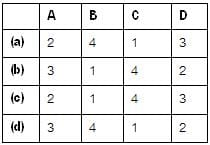Test: Circuit Theorems- 2 - Question 4

The v-i characteristic of an element is shown in figure below. The element is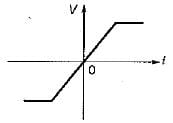Test: Circuit Theorems- 2 - Question 5

If the source impedance is inductive, for maximum transfer of power from the source to the load, the load should be

Test: Circuit Theorems- 2 - Question 6

The equivalent resistance between terminal A and B for the given circuit is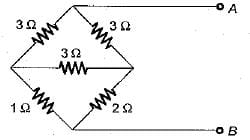Detailed Solution for Test: Circuit Theorems- 2 - Question 6

Converting 3 Ω delta connected resistors into star-equivalent, the circuit is reduced as shown below.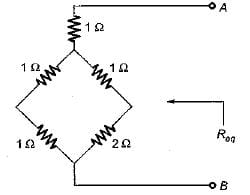∴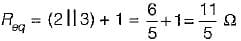Test: Circuit Theorems- 2 - Question 7

Assertion (A): Norton’s theorem is called dual of the Thevenin’s theorem.
Reason (R): If the Thevenin’s equivalent voltage source is converted to an equivalent current source, the Norton’s equivalent is obtained.

Test: Circuit Theorems- 2 - Question 8

Assertion (A): In any active network, maximum power transfer to the load takes place when the load impedance is equal to an equivalent impedance of the network as viewed from the terminals of the load.
Reason (R): For finding the maximum power transfer to the load, any network has to be first converted into Th even in’s equivalent across the terminals of the load.

Detailed Solution for Test: Circuit Theorems- 2 - Question 8

ZL = Z*S for maximum power transfer. Hence, assertion is a false statement.

Test: Circuit Theorems- 2 - Question 9

Assertion (A): The substitution theorem is very useful in the networks consisting the mutual inductances.
Reason (R): By the substitution theorem, the effect of mutual inductances may be replaced by equivalent dependent sources of the same Value.

Test: Circuit Theorems- 2 - Question 10

Consider the following statements related to
Thevenin’s theorem:
For the application of Thevenin’s theorem
1. the circuit should not. contain non-iinear elements.
2. the circuit may contain unilateral or bilateral elements or both.
3. there should not be magnetic coupling between the load and circuit, to be replaced by Thevenin's theorem.
4. there should not be controlled sources on the load size, controlled from some other oart of the circuit.

## Topicwise Question Bank for Electrical Engineering

211 tests
Information about Test: Circuit Theorems- 2 Page
In this test you can find the Exam questions for Test: Circuit Theorems- 2 solved & explained in the simplest way possible. Besides giving Questions and answers for Test: Circuit Theorems- 2, EduRev gives you an ample number of Online tests for practice

## Topicwise Question Bank for Electrical Engineering

211 tests(Scan QR code)